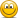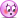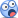Errors Found in Study Materials P1.T3. Financial Markets & Products (OLD thread)

Status
Not open for further replies.

David Harper CFA FRM

David Harper CFA FRM
Staff member
Subscriber
Hi @Elizabeth_Babalola Thank you, I hope you are keeping safe also!Can I clarify: do you mean that, in the denominator of the formula, instead of 1+Rf(T2 - T1), it should read 1+(Rf(T2 - T1))? And/or do you mean, in the equivalent calculation that, instead of (1+ 4.5 × 025), it should read (1+ (4.5 × 025))? If so, it's actually not necessary per order of operations (https://en.wikipedia.org/wiki/Order_of_operations) where multiplication is performed before addition. I do realize the calculator sets either way, but in mathematical expression, we (aka, everybody and commonly) rely on order of operations. Further, I think it's okay to expect the reader to understand that the discounting is with quarterly frequency is what's happening here; i.e., 1 + Rf*0.25 = (1 + Rf/k)^(T*k) in the case of k = 4 when T - 0.25 --> (1 + Rf/4)^(025*4) = (1 + Rf/4)^1 = (1 + Rf * 0.25). We should understand this is not annual compounding/discounting but rather discounting with quarterly compound frequency, if you like. If I misunderstood the correct requested, apologies?

Last edited:

Elizabeth_Babalola

Member
I am well too.I guess i didn't meet the expectation. i literally spent a while punching my calculator. It could be that i lost touch.

Sadly, it's been a while i read. @David Harper CFA FRM, thanks for clarifying.

David Harper CFA FRM

David Harper CFA FRM
Staff member
Subscriber
No worries! It would not be wrong to add the parens, but if we had to do that in every instance (to reinforce the order of ops), we'd have to redo a lot of formulas!Talk to you soon!

David Harper CFA FRM

David Harper CFA FRM
Staff member
Subscriber
Hi @Elizabeth_Babalola My apologies, but that is a mistake. (Thank you!). It should be that either:
• Solution given (follows text) is: 0.98*exp[(0.070 - 0.050)*2) = 1.020; or
• Question setup (follows solutions) is: "Suppose that the 2-year interest rates in Australia and the United States are 3% and 1%, respectively, and the spot exchange rate is 0.9800 USD per AUD."

David Harper CFA FRM

David Harper CFA FRM
Staff member
Subscriber
@Elizabeth_Babalola Yes, good catch! Our mistake. The total gain is the difference of differences: (5.2% - 4.0%) - (L + 0.6%) - (L - 0.1%) = 1.2% - 0.7% = 0.50%. Thank you for spotting this mistake

jan_science17

New Member
Hi David!

Im a bit confused. With regard to the value of forward contracts, do we always have to bring to its present value? As given in the formula on the study notes Chapter 10 page 7.

However, on the given example on page 8, particularly with the Ex. 5.4: Forward value of the stock, the amount was its future value and the negative sign was not taken into consideration in the computation??Thanks for clarification!

Regards,
Jan

P.S. Re. with my above question, the word "value" should be replaced by "price" since the computation pertains to the F(0) of the stock and the related formula should also be edited to remove the "-" (negative sign)? Thanks! - Jan

David Harper CFA FRM

David Harper CFA FRM
Staff member
Subscriber
Hi David!

Im a bit confused. With regard to the value of forward contracts, do we always have to bring to its present value? As given in the formula on the study notes Chapter 10 page 7.

However, on the given example on page 8, particularly with the Ex. 5.4: Forward value of the stock, the amount was its future value and the negative sign was not taken into consideration in the computation??

View attachment 2863

Thanks for clarification!

Regards,
Jan

P.S. Re. with my above question, the word "value" should be replaced by "price" since the computation pertains to the F(0) of the stock and the related formula should also be edited to remove the "-" (negative sign)? Thanks! - Jan

Hi @jan_science17 You are right to be confused, it's my mistake. The Ex 5.4. equation, in order to match the formula displayed as 25*exp(10%*0.5) = $26.28, and to also match the surrounding cost-of-carry (COC) instances, should read F(0) = S(0)*exp(r*T). Its a giveway (hint) that the formula is "out of place" because it solves for smaller case "f", which here denotes present value, rather than "F(0)" which denotes a forward price. To answer your question, "With regard to the value of forward contracts, do we always have to bring to its present value?", no we do not. Where might we want to estimate future value? When estimating counterparty credit risk (Part 2, Topic 6) where contracts have longer terms (beyond one year), we are often interested in the "expected future value." This is even more true of prices, although due to connotations, personally I do not hinge myself to a hard distinction between price and value. I think many would say that price as something that is observed (e.g., traded) while value is theoretical (e.g., intrinsic value of a stock)--as in "price is what you pay, value is what you get"--however in finance we are very often computing the theoretical price of something .... In this context, which is all based in Hull and follows Hull's notation, the key variables w.r.t. the forward contract are the the spot price, S(0), the delivery (aka, strike) price, K, the forward price, F(0), and the value of the forward contract, denoted lower case f. When these are understood, I think it's good to see why the delivery price, K, does not need a subscript (the strike is fixed over the life); why F(0) is a vector of several current forward prices such that F(0,T) is good alternative notation; and why f does not need a subscript because it connotes present value but there is nothing wrong with f(T) which estimates a future value of the contract. Let's say the current spot price, S(0) =$25.00 and the asset is a non-dividend-paying stock so the COC model, which prices the forward, tells us that the theoretical forward price for a 0.5-year contract is F(0) = S(0)*exp(rT) = $25.00*exp(10%*0.5) =$26.28; i.e., our note should read "Ex 5.4. Forward price of stock; F(0) = S(0)*exp(rT); $25.00*exp(10%*0.5) =$26.28". It's a theoretical price; we aren't surprised if the actual contract trades rich/cheap due to factors outside the model. But if a new forward contract follows the theoretical price, then a new contract has a delivery price (in six months) of $26.28 such that K =$26.28. That's a contract, at inception, with zero value: at inception, we assume K = F(0) because that's the delivery price, K, that gives the contract an immediate value of zero.

If the stock grows at the riskfree rate, we might expect future S(t) = S(0)*exp(rT). Then the future value of (long position in) the forward contract is estimated to be S(t) - K = [S(0)*exp(rT)] - K. And the present value of this future value is f(0) = f = [S(0)*exp(rT) - K]*exp(-rT) = S(0) - K*exp(-rT). This value is zero at inception but imagine we go forward three months and the stock price increases to $35.00. The value of the contract will be, f =$35 - $25*exp(-10%*0.25) =$10.62. The strike, K, does not change, but the value fluctuates. My reply is a longer than i intended but I hope it's helpful!

Last edited:

yashitrivedi

New Member
@David Harper CFA FRM @Nicole Seaman I'm looking at page 9 of study notes for the chapter "Exchanges and OTC Markets" at the example on complete clearing. The values for A and C when clearing with a counterparty seem to be incorrect. I've highlighted in yellow the values I feel are incorrect and suggested the correct values in red. Keen to know your thoughts. Thanks!yashitrivedi

New Member
@David Harper CFA FRM @Nicole Seaman One more coming again from the chapter "Exchanges and OTC Markets". This one's on page 20 of the study notes where different risks are being outlined associated with each of the derivative products. For commodities, the notes mention that there may be a potential pricing risk but the summary table below does not say "yes" for this. I'm assuming the typo is in the table and it should have been a Yes there.Nicole Seaman

Director of FRM Operations
Staff member
Subscriber
Status
Not open for further replies.